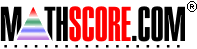Math Practice Online > free > lessons > Florida > 6th grade > Rounding Numbers

## Rounding Numbers

Practice rounding integers from 1 to 10,000

 Sample Problems for Rounding Numbers Lesson for Rounding Numbers

### This topic aligns to the following state standards

Grade 3: Num 1. Uses estimation strategies to determine a reasonable estimate of a quantity.
Grade 4: Num 1. Chooses, describes and explains estimation strategies used to determine the reasonableness of solutions to real-world problems.
Grade 5: Num 1. Chooses, describes, and explains estimation strategies used to determine the reasonableness of solutions to real-world problems.

Copyright Accurate Learning Systems Corporation 2008.